## Time Variance

To compute variance of a large measurement sequence data, recursive time-variance formula (4) below give more efficient computation and data storage. You don't need to keep all the measurement data. All you need is to compute the current variance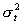is the current measurement data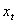, current time-average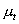and the previous variance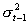. The formula is as follow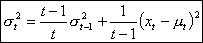(4)

For time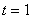, it is defined that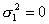. Thus, the computation using equation (4) starts at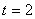.

Using the previous measurement data of 4, 6, 12, 9 we can get exactly the same result of variance as the usual computation method, but in more efficient way. Table below show the example of computation using the recursive time-variance formula above.

 Time (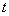) Measurement () Average () Variance () 1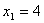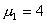(by definition). 2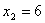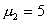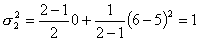3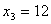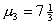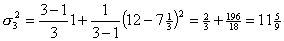4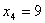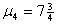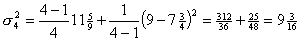To give you better understanding about recursive mean and variance, I made a simple program below. You can try with your own data or test it with the example above. To use the program you need to set JavaScript enabled in your browser and you need to type the input data one at a time (I include 4 as an initial current input data), then press "Input" button to compute the time average and time variance. Alternatively, click "Random Input" button repeatedly to create simulated data. You need at least two data points to plot the chart. To restart with an empty data, click "Reset" button. If you use Internet Explorer, the chart may not work properly to show the effect of variance, please use FireFox or Google Chrome instead.

Current input data

Current Average:
0

Current Variance:
0

Previous Average:
0

Previous Variance:
0

Number of data:
0